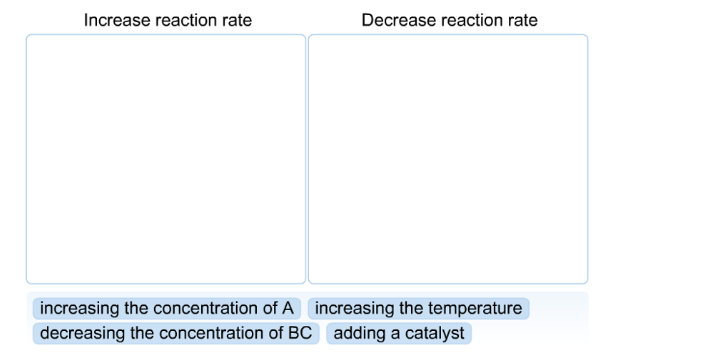Problem: How would the following events affect the rate of the following general reaction?A + BC → ABC

79% (91 ratings)
Problem Details

How would the following events affect the rate of the following general reaction?

A + BC → ABCWhat scientific concept do you need to know in order to solve this problem?

Our tutors have indicated that to solve this problem you will need to apply the Intro to Chemical Kinetics concept. You can view video lessons to learn Intro to Chemical Kinetics Or if you need more Intro to Chemical Kinetics practice, you can also practice Intro to Chemical Kinetics practice problems .

What is the difficulty of this problem?

Our tutors rated the difficulty of How would the following events affect the rate of the follow... as medium difficulty.

How long does this problem take to solve?

Our expert Chemistry tutor, Sabrina took 3 minutes to solve this problem. You can follow their steps in the video explanation above.

What professor is this problem relevant for?

Based on our data, we think this problem is relevant for Professor Orgill's class at UNLV.